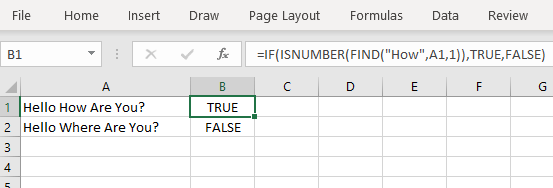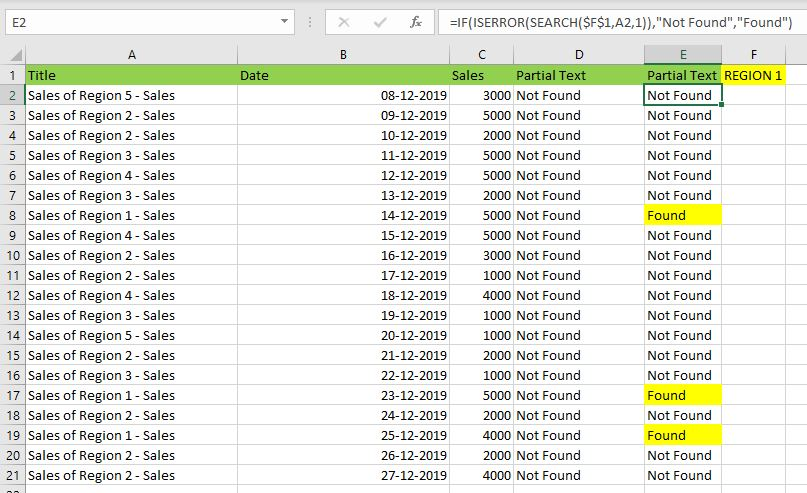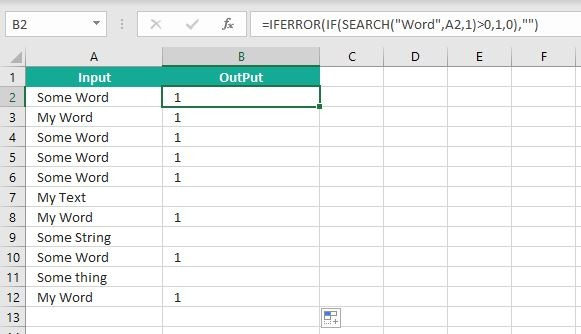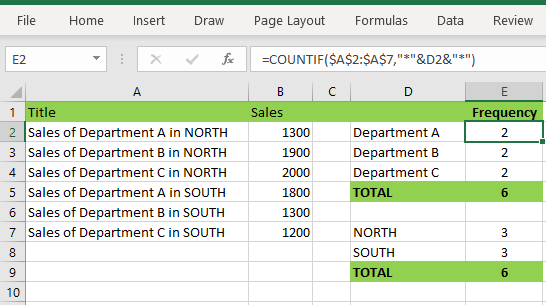Excel If Cell Contains Text Then Formula helps you to return the output when a cell have any text or a specific text. You can check if a cell contains a some string or text  and produce something in other cell. For Example you can check if a cell A1 contains text ‘example text’  and print Yes or No in Cell B1. Following are the example Formulas to check if Cell contains text then return some thing in a Cell.

## If Cell Contains Text

Here are the Excel formulas to check if Cell contains specific text then return something. This will return if there is any string or any text in given Cell. We can use this simple approach to check if a cell contains text, specific text, string,  any text using Excel If formula. We can use equals to  operator(=) to compare the strings .

### If Cell Contains Text Then TRUE

Following is the Excel formula to return True if a Cell contains Specif Text. You can check a cell if there is given string in the Cell and return True or False.

=IF(ISNUMBER(FIND(“How”,A1,1)),TRUE,FALSE)

The formula will return true if it found the match, returns False of no match found.### If Cell Contains Partial Text

We can return Text If Cell Contains Partial Text. We use formula or VBA to Check Partial Text in a Cell.

#### Find for Case Sensitive Match:

We can check if a Cell Contains Partial Text then return something using Excel Formula. Following is a simple example to find the partial text in a given Cell. We can use if your want to make the criteria case sensitive.• Here, Find Function returns the finding position of the given string
• Use Find function is Case Sensitive
• IsError Function check if Find Function returns Error, that means, string not found

#### Search for Not Case Sensitive Match:

We can use Search function to check if Cell Contains Partial Text. Search function useful if you want to make the checking criteria Not Case Sensitive.### If Range of Cells Contains Text

We can check for the strings in a range of cells. Here is the formula to find If Range of Cells Contains Text. We can use Count If Formula to check the excel if range of cells contains specific text and return Text.

=IF(COUNTIF(A2:A21, “*Region 1d*”)>0,”Range Contais Text”,”Text Not Found in the Given Range”)
• CountIf function counts the number of cells with given criteria
• We can use If function to return the required Text
• Formula displays the Text ‘Range Contains Text” if match found
• Returns “Text Not Found in the Given Range” if match not found in the specified range

### If Cells Contains Text From List

Below formulas returns text If Cells Contains Text from given List. You can use based on your requirement.

VlookUp to Check If Cell Contains Text from a List:
We can use VlookUp function to match the text in the Given list of Cells. And return the corresponding values.

• Check if a List Contains Text:
=IF(ISERR(VLOOKUP(F1,A1:B21,2,FALSE)),”False:Not Contains”,”True: Text Found”)
• Check if a List Contains Text and Return Corresponding Value:
=VLOOKUP(F1,A1:B21,2,FALSE)
• Check if a List Contains Partial Text and Return its Value:
=VLOOKUP(“*”&F1&”*”,A1:B21,2,FALSE)

### If Cell Contains Text Then Return a Value

We can return some value if cell contains some string. Here is the the the Excel formula to return a value if a Cell contains Text. You can check a cell if there is given string in the Cell and return some string or value in another column.The formula will return true if it found the match, returns False of no match found. can

### Excel if cell contains word then assign value

You can replace any word in the following formula to check if cell contains word then assign value.

=IFERROR(IF(SEARCH(“Word”,A2,1)>0,1,0),””)Search function will check for a given word in the required cell and return it’s position. We can use If function to check if the value is greater than 0 and assign a given value (example: 1) in the cell. search function returns #Value if there is no match found in the cell, we can handle this using IFERROR function.

### Count If Cell Contains Text

We can check If Cell Contains Text Then COUNT. Here is the Excel formula to Count if a Cell contains Text. You can count the number of cells containing specific text.

=COUNTIF(\$A\$2:\$A\$7,”*”&D2&”*”)

The formula will Sum the values in Column B if the cells of Column A contains the given text.### Count If Cell Contains Partial Text

We can count the cells based on partial match criteria. The following Excel formula Counts if a Cell contains Partial Text.

=COUNTIF(A2:A21, “*Region 1*”)
• We can use the CountIf Function to Count the Cells if they contains given String
• Wild-card operators helps to make the CountIf to check for the Partial String
• Put Your Text between two asterisk symbols (*YourText*) to make the criteria to find any where in the given Cell
• Add Asterisk symbol at end of your text (YourText*) to make the criteria to find your text beginning of given Cell
• Place Asterisk symbol at beginning of your text (*YourText) to make the criteria to find your text end of given Cell

### If Cell contains text from list then return value

Here is the Excel Formula to check if cell contains text from list then return value. We can use COUNTIF and OR function to check the array of values in a Cell and return the given Value. Here is the formula to check the list in range D2:D5 and check in Cell A2 and return value in B2.

=IF(OR(COUNTIF(A2,”*”&\$D\$2:\$D\$5&”*”)), “Return Value”, “”)### If Cell Contains Text Then SUM

Following is the Excel formula to Sum if a Cell contains Text. You can total the cell values if there is given string in the Cell. Here is the example to sum the column B values based on the values in another Column.

=SUMIF(\$A\$2:\$A\$7,”*”&D2&”*”,\$B\$2:\$B\$7)

The formula will Sum the values in Column B if the cells of Column A contains the given text.### Sum If Cell Contains Partial Text

Use SumIfs function to Sum the cells based on partial match criteria. The following Excel formula Sums the Values if a Cell contains Partial Text.

=SUMIFS(C2:C21,A2:A21, “*Region 1*”)
• SUMIFS Function will Sum the Given Sum Range
• We can specify the Criteria Range, and wild-card expression to check for the Partial text
• Put Your Text between two asterisk symbols (*YourText*) to Sum the Cells if the criteria to find any where in the given Cell
• Add Asterisk symbol at end of your text (YourText*) to Sum the Cells if the criteria to find your text beginning of given Cell
• Place Asterisk symbol at beginning of your text (*YourText) to Sum the Cells if criteria to find your text end of given Cell

### VBA to check if Cell Contains Text

Here is the VBA function to find If Cells Contains Text using Excel VBA Macros.

#### If Cell Contains Partial Text VBA

We can use VBA to check if Cell Contains Text and Return Value. Here is the simple VBA code match the partial text. Excel VBA if Cell contains partial text macros helps you to use in your procedures and functions.

Sub sbCkeckforPartialText()
MsgBox CheckIfCellContainsPartialText(Cells(2, 1), “Region 1”)
End Sub
Function CheckIfCellContainsPartialText(ByVal cell As Range, ByVal strText As String) As Boolean
If InStr(1, cell.Value, strText) > 0 Then CheckIfCellContainsPartialText = True
End Function
• CheckIfCellContainsPartialText VBA Function returns true if Cell Contains Partial Text
• inStr Function will return the Match Position in the given string

#### If Cell Contains Text Then VBA MsgBox

Here is the simple VBA code to display message box if cell contains text. We can use inStr Function to search for the given string. And show the required message to the user.

Sub sbVBAIfCellsContainsText()
If InStr(1, Cells(2, 1), “Region 3”) > 0 Then blnMatch = True
If blnMatch = True Then MsgBox “Cell Contains Text”
End Sub
• inStr Function will return the Match Position in the given string
• blnMatch is the Boolean variable becomes True when match string
• You can display the message to the user if a Range Contains Text

#### Which function returns true if cell a1 contains text?

You can use the Excel If function and Find function to return TRUE if Cell A1 Contains Text. Here is the formula to return True.

=IF(ISNUMBER(FIND(“YourText”,A1,1)),TRUE,FALSE)

#### Which function returns true if cell a1 contains text value?

You can use the Excel If function with Find function to return TRUE if a Cell A1 Contains Text Value. Below is the formula to return True based on the text value.

=IF(ISNUMBER(FIND(“YourTextValue”,A1,1)),TRUE,FALSE)

1. Meghana December 27, 2019 at 1:42 pm - Reply

Hi Sir,Thank you for the great explanation, covers everything and helps use create formulas if cell contains text values.

Many thanks! Meghana!!

2. Max December 27, 2019 at 4:44 pm - Reply

Perfect! Very Simple and Clear explanation. Thanks!!

3. Mike Song August 29, 2022 at 2:45 pm - Reply

I tried this exact formula and it did not work.